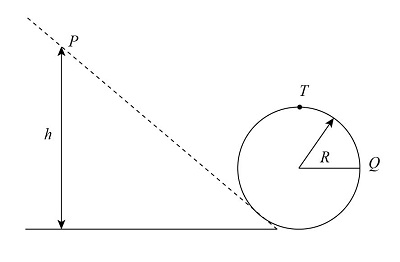# A solid brass ball of mass 9.5 g will roll smoothly along a loop-the-loop track when released...

## Question:

A solid brass ball of mass 9.5 g will roll smoothly along a loop-the-loop track when released from rest along the straight section. The circular loop has radius R = 4.2 m, and the ball has radius r << R.

(a) What is h if the ball is on the verge of leaving the track when it reaches the top of the loop?

(b) If the ball is released at height h = 6.00R, what is the magnitude of the horizontal force component acting on the ball at point Q?

## Potential energy:

The energy of an object under some specific height at which the object is placed is called the potential energy of an object. Mathematically it is expressed as the multiplication of mass , height, and the gravitational acceleration on the object.

Given data:

• mass of ball is, {eq}m = 9.5\;{\rm{g}} {/eq}.
• radius of loop is, {eq}R = 4.2\;m {/eq}.(a) Applying the law of conservation of energy at P and T in the above figure as,

{eq}{K_P} + {U_P} = {K_T} + {U_T} {/eq}

Here, K and U are the kinetic and potential energy respectively.

{eq}0 + mgh = \dfrac{1}{2}I{\omega ^2} + \dfrac{1}{2}m{V^2} + mg\left( {2R} \right) {/eq}

Here, I is the moment of inertia about the center of mass of the ball. And for the circular section,

{eq}I = \dfrac{2}{5}m{R^2} {/eq}. And {eq}\omega {/eq} is the rotational speed of ball.

Solve by substituting the values in above equation as,

{eq}\begin{align*} mgh &= \dfrac{1}{2}\left( {\dfrac{2}{5}m{R^2}} \right){\omega ^2} + \dfrac{1}{2}m{R^2}{\omega ^2} + 2mgR\\ mgh &= \dfrac{7}{{10}}m{R^2}{\omega ^2} + 2mgR\\ gh &= \dfrac{7}{{10}}{V^2} + 2gR.............................\left( 1 \right) \end{align*} {/eq}

Velocity of the ball at the top is,

{eq}V = \sqrt {g\left( {R - r} \right)} {/eq}

Since r<< R.

Then,

{eq}V = \sqrt {gR} {/eq}

Substituting the above value in equation 1 as,

{eq}\begin{align*} gh &= \dfrac{7}{{10}}gR + 2gR\\ h &= \left( {\dfrac{7}{{10}} + 2} \right)R\\ h &= 2.7 \times 4.2\\ h &= 11.34\;{\rm{m}} \end{align*} {/eq}

Thus, the value for h is 11.34 m.

(b) Applying the law of conservation of energy at Q and P as,

{eq}\begin{align*} {K_P} + {U_P} = {K_Q} + {U_P}\\ 0 + mgh = \dfrac{1}{2}I{\omega ^2} + \dfrac{1}{2}m{V^2} + mg\left( R \right) \end{align*} {/eq}

Solving further as,

{eq}\begin{align*} mgh &= \dfrac{1}{2}\left( {\dfrac{2}{5}m{R^2}} \right){\omega ^2} + \dfrac{1}{2}m{R^2}{\omega ^2} + mgR\\ mgh &= \dfrac{7}{{10}}m{R^2}{\omega ^2} + mgR\\ gh &= \dfrac{7}{{10}}{V^2} + gR.............................\left( 2 \right) \end{align*} {/eq}

Since h = 6R,

Substituting the value in the equation 2 as,

{eq}\begin{align*} g\left( {6R} \right) &= \dfrac{7}{{10}}{V^2} + gR\\ 5gR &= \dfrac{7}{{10}}{V^2}\\ {V^2} &= \dfrac{{50gR}}{7} \end{align*} {/eq}

The horizontal force on ball at Q is,

{eq}\begin{align*} F &= ma\\ F &= m\dfrac{{{V^2}}}{{\left( {R - r} \right)}} \end{align*} {/eq}

Since r<<<R .

{eq}F = m\dfrac{{{V^2}}}{R} {/eq}

Solve by substituting the values in the above expression as,

{eq}\begin{align*} F &= 0.0095 \times \dfrac{{50gR}}{7} \times \dfrac{1}{R}\\ F &= 0.0095 \times \dfrac{{50 \times 9.8 \times 4.2}}{7}\\ F &= 2.79\;{\rm{N}} \end{align*} {/eq}

Thus, the horizontal force on the ball at Q is 2.79 N.